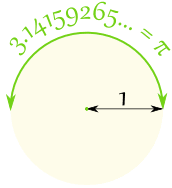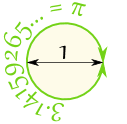# Pi (π)Draw a circle with a radius of 1. The distance half way around the edge of the circle will be 3,14159265... a number known as Pi
 Or you could draw a circle with a diameter of 1. Then the circumference (the distance all the way around the edge of the circle) will be PiPi (the symbol is the Greek letter π) is: The ratio of the Circumference  to the Diameter  of a Circle.   Area of Circle = π r2 Circumference of a Circle = 2π r